# Modeling

## Introduction

#### What

Can explain models

A model is a representation of something else.

A class diagram is a model that represents a software design.

A model provides a simpler view of a complex entity because a model captures only a selected aspect. This omission of some aspects implies models are abstractions.

A class diagram captures the structure of the software design but not the behavior.

Multiple models of the same entity may be needed to capture it fully.

In addition to a class diagram (or even multiple class diagrams), a number of other diagrams may be needed to capture various interesting aspects of the software.

#### How

Can explain how models are used

In software development, models are useful in several ways:

a) To analyze a complex entity related to software development.

Some examples of using models for analysis:

1. Models of the can be built to aid the understanding of the problem to be solved.
2. When planning a software solution, models can be created to figure out how the solution is to be built. An architecture diagram is such a model.

b) To communicate information among stakeholders. Models can be used as a visual aid in discussions and documentation.

Some examples of using models to communicate:

1. You can use an architecture diagram to explain the high-level design of the software to developers.
2. A business analyst can use a use case diagram to explain to the customer the functionality of the system.
3. A class diagram can be reverse-engineered from code so as to help explain the design of a component to a new developer.

c) As a blueprint for creating software. Models can be used as instructions for building software.

Some examples of using models as blueprints:

1. A senior developer draws a class diagram to propose a design for an OOP software and passes it to a junior programmer to implement.
2. A software tool allows users to draw UML models using its interface and the tool automatically generates the code based on the model.
Model Driven Development extra

Exercises

#### UML models

Can identify UML models

The following diagram uses the class diagram notation to show the different types of UML diagrams.source:https://en.wikipedia.org/

## Modeling structures

#### OO structures

Can explain structure modeling of OO solutions

An OO solution is basically a network of objects interacting with each other. Therefore, it is useful to be able to model how the relevant objects are 'networked' together inside a software i.e. how the objects are connected together.

Given below is an illustration of some objects and how they are connected together. Note: the diagram uses an ad-hoc notation.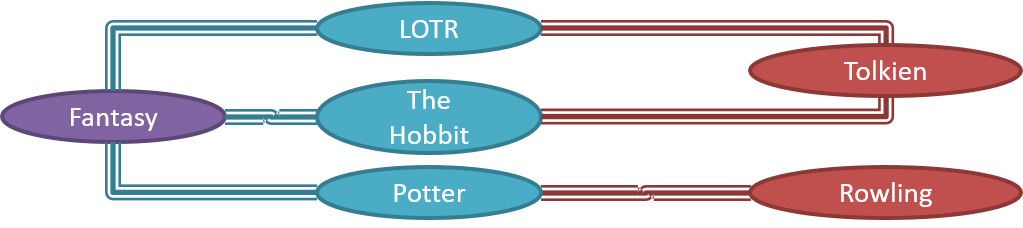Note that these object structures within the same software can change over time.

Given below is how the object structure in the previous example could have looked like at a different time.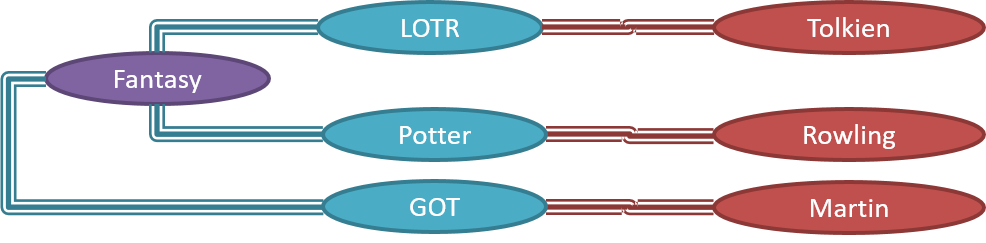However, object structures do not change at random; they change based on a set of rules, as was decided by the designer of that software. Those rules that object structures need to follow can be illustrated as a class structure i.e. a structure that exists among the relevant classes.

Here is a class structure (drawn using an ad-hoc notation) that matches the object structures given in the previous two examples. For example, note how this class structure does not allow any connection between `Genre` objects and `Author` objects, a rule followed by the two object structures above.UML Object Diagrams are used to model object structures and UML Class Diagrams are used to model class structures of an OO solution.

Here is an object diagram for the above example: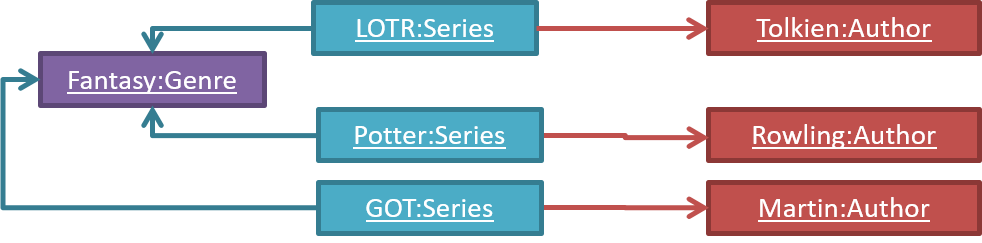And here is the class diagram for it: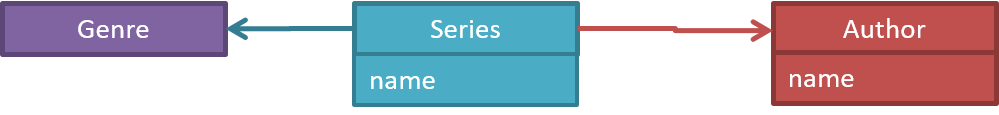#### Class diagrams - basic

Can use basic-level class diagrams

Contents of the panels given below belong to a different chapter; they have been embedded here for convenience and are collapsed by default to avoid content duplication in the printed version.

UML Class Diagrams → Introduction → What

Classes form the basis of class diagrams.

UML Class Diagrams → Classes → What

UML Class Diagrams → Class-Level Members → What

Associations are the main connections among the classes in a class diagram.

OOP Associations → What

UML Class Diagrams → Associations → What

The most basic class diagram is a bunch of classes with some solid lines among them to represent associations, such as this one.

An example class diagram showing associations between classes.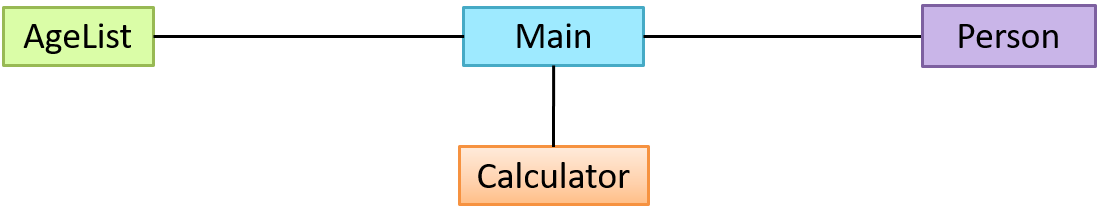UML Class Diagrams → Associations → Labels

UML Class Diagrams → Associations → Roles

OOP Associations → Multiplicity

UML Class Diagrams → Associations → Multiplicity

OOP Associations → Navigability

UML Class Diagrams → Associations → Navigability

Here is the same class diagram shown earlier but with some additional information included: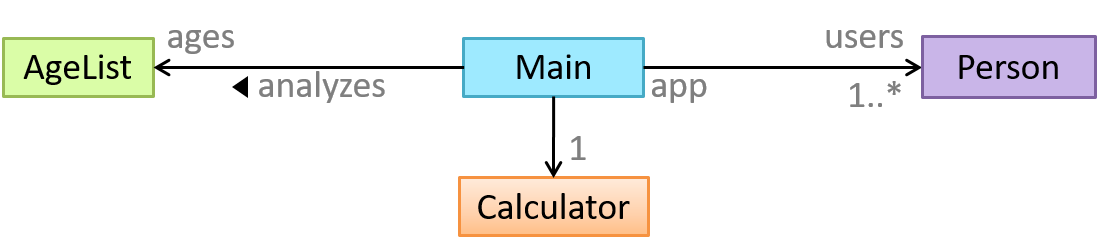Exercises

Which association notations are shown?

Explain Class Diagram

Draw Class Diagram for Box etc.

UML → Notes

#### Class diagrams - intermediate

Can use intermediate-level class diagrams

A class diagram can also show different types of relationships between classes: inheritance, compositions, aggregations, dependencies.

##### Modeling inheritance

OOP → Inheritance → What

UML → Class Diagrams → Inheritance → What

##### Modeling composition

OOP → Associations → Composition

UML → Class Diagrams → Composition → What

##### Modeling aggregation

OOP → Associations → Aggregation

UML → Class Diagrams → Aggregation → What

##### Modeling dependencies

OOP → Associations → Dependencies

UML → Class Diagrams → Dependencies → What

A class diagram can also show different types of class-like entities:

##### Modeling enumerations

OOP → Classes → Enumerations

UML → Class Diagrams → Enumerations → What

##### Modeling abstract classes

OOP → Inheritance → Abstract Classes

UML → Class Diagrams → Abstract Classes → What

##### Modeling interfaces

OOP → Inheritance → Interfaces

UML → Class Diagrams → Interfaces → What

Exercises

Explain notations in the class diagram

Draw a Class Diagram for the code (`StockItem`, `Inventory`, `Review`, etc.)

A class diagram can show association classes too.

OOP → Associations → Association Classes

UML → Class Diagrams → Association Classes → What

Exercises

#### Object diagrams

Can use basic object diagrams

UML → Object Diagrams → Introduction

Object diagrams can be used to complement class diagrams. For example, you can use object diagrams to model different object structures that can result from a design represented by a given class diagram.

UML → Object Diagrams → Objects

UML → Object Diagrams → Associations

Exercises

Draw an Object Diagram for Box etc.

#### Object oriented domain models

Can explain object oriented domain models

The analysis process for identifying objects and object classes is recognized as one of the most difficult areas of object-oriented development. --Ian Sommerville, in the book Software Engineering

Class diagrams can also be used to model objects in the (i.e. to model how objects actually interact in the real world, before emulating them in the solution). Class diagrams that are used to model the problem domain are called conceptual class diagrams or OO domain models (OODMs).

The OO domain model of a snakes and ladders game is given below.

Description: The snakes and ladders game is played by two or more players using a board and a die. The board has 100 squares marked 1 to 100. Each player owns one piece. Players take turns to throw the die and advance their piece by the number of squares they earned from the die throw. The board has a number of snakes. If a player’s piece lands on a square with a snake head, the piece is automatically moved to the square containing the snake’s tail. Similarly, a piece can automatically move from a ladder foot to the ladder top. The player whose piece is the first to reach the 100th square wins.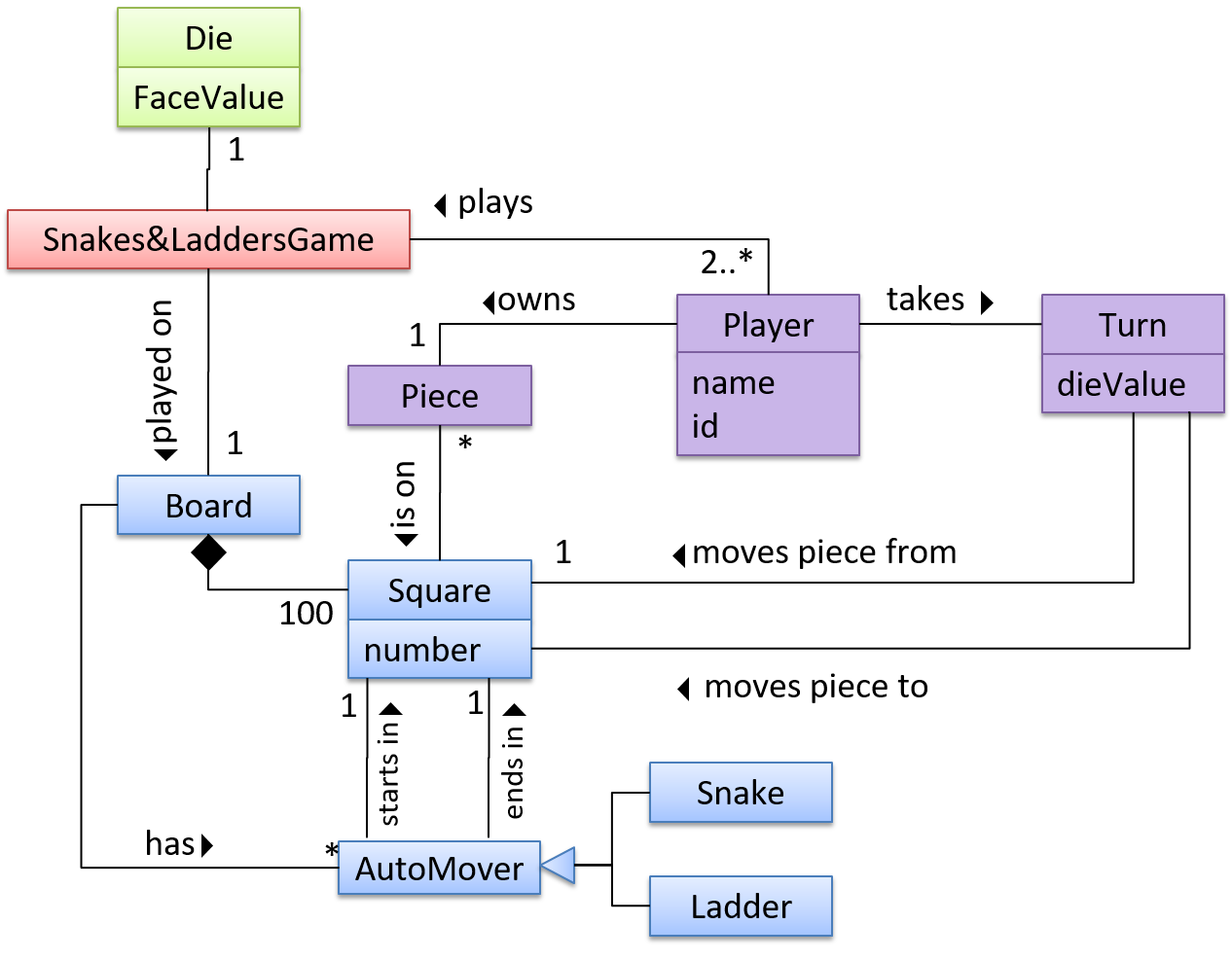OODMs do not contain solution-specific classes (i.e. classes that are used in the solution domain but do not exist in the problem domain). For example, a class called DatabaseConnection could appear in a class diagram but not usually in an OO domain model because DatabaseConnection is something related to a software solution but not an entity in the problem domain.

OODMs represents the class structure of the problem domain and not their behavior, just like class diagrams. To show behavior, use other diagrams such as sequence diagrams.

OODM notation is similar to class diagram notation but omit methods and navigability.

Exercises

This diagram is,...

Difference between a class diagram and an OO domain model?

#### Deployment diagrams

Can explain deployment diagrams

A deployment diagram shows a system's physical layout, revealing which pieces of software run on which pieces of hardware.

An example deployment diagram: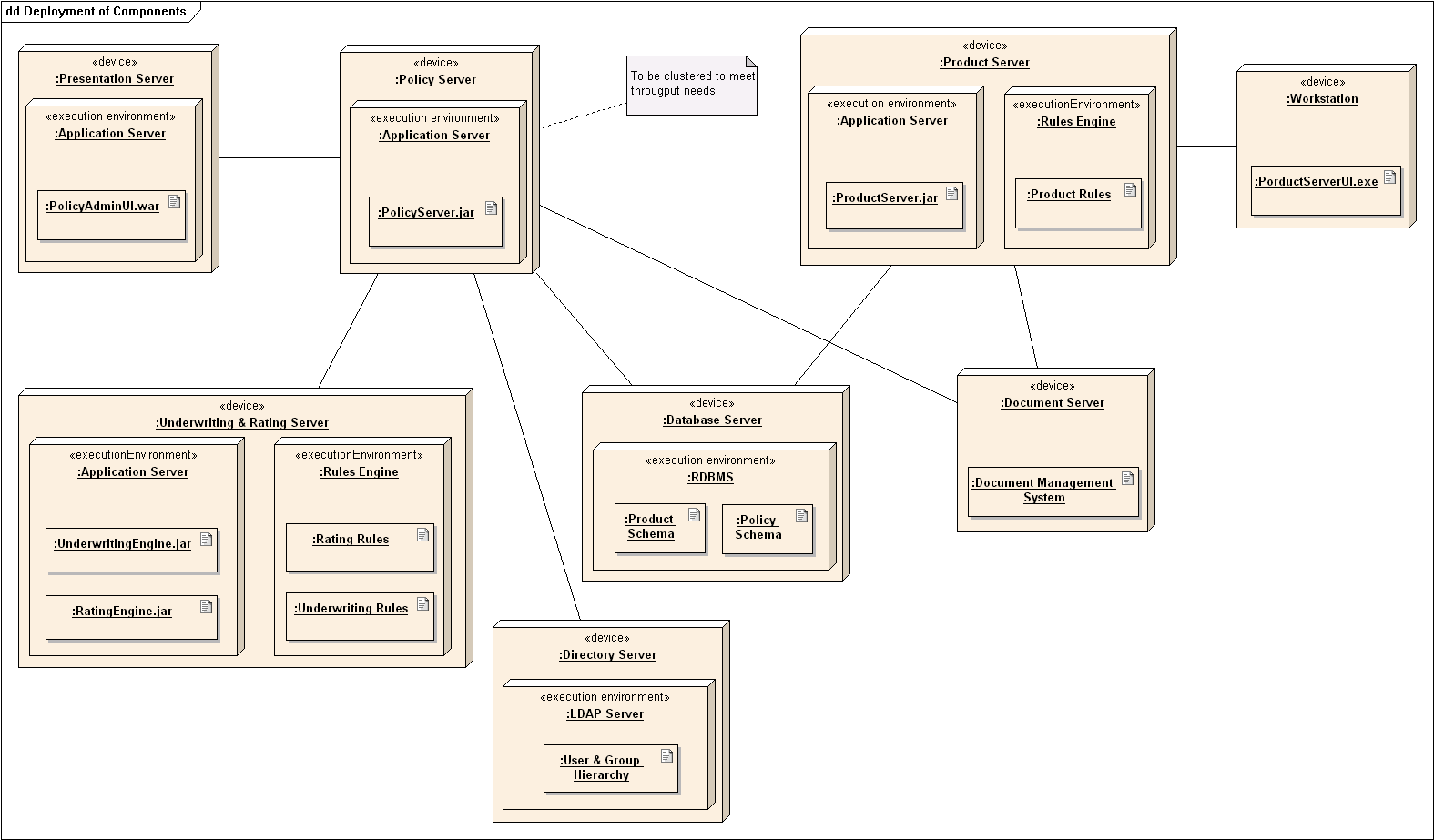source:https://commons.wikimedia.org

#### Component diagrams

Can explain component diagrams

A component diagram is used to show how a system is divided into components and how they are connected to each other through interfaces.

An example component diagram: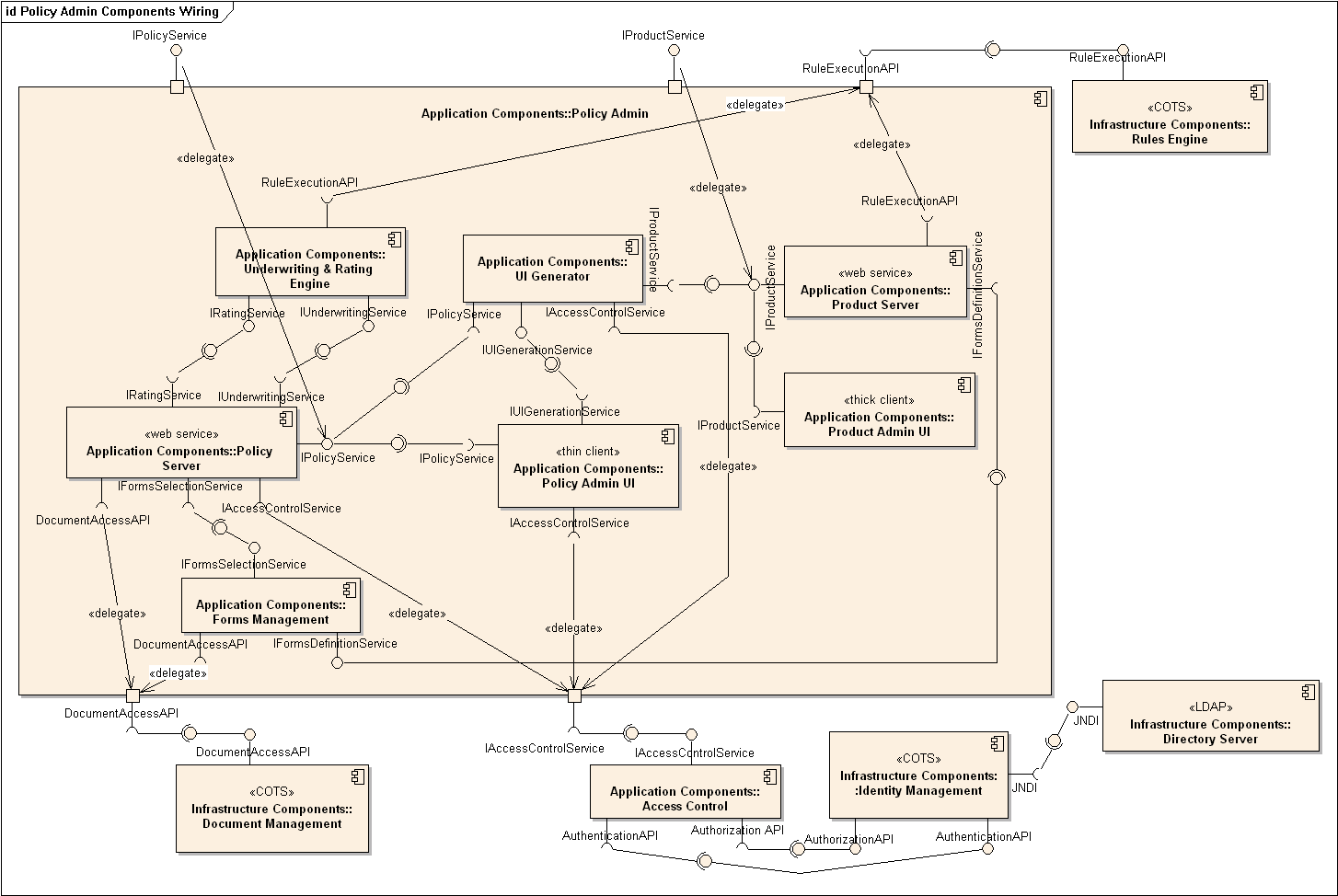source:https://commons.wikimedia.org

#### Package diagrams

Can explain package diagrams

A package diagram shows packages and their dependencies. A package is a grouping construct for grouping UML elements (classes, use cases, etc.).

Here is an example package diagram: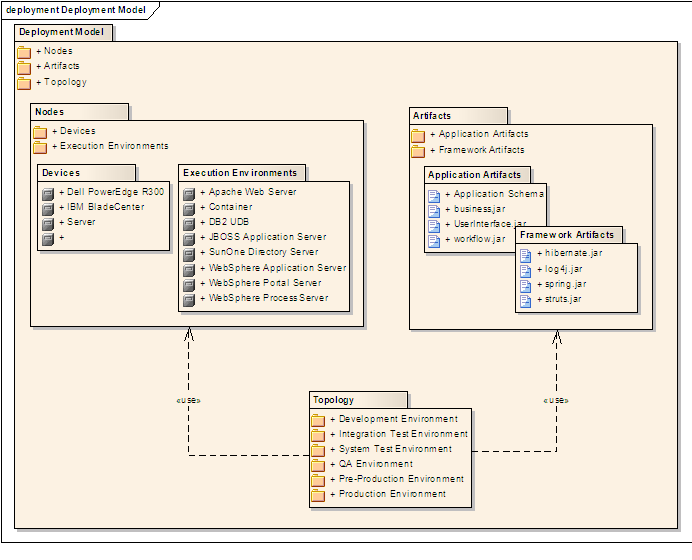source:https://commons.wikimedia.org

#### Composite structure diagrams

Can explain composite structure diagrams

A composite structure diagram hierarchically decomposes a class into its internal structure.

Here is an example composite structure diagram: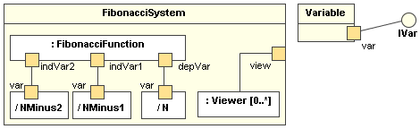source:https://commons.wikimedia.org

## Modeling behaviors

#### Activity diagrams - basic

Can use basic-level activity diagrams

Software projects often involve workflows. Workflows define the flow in which a process or a set of tasks is executed. Understanding such workflows is important for the success of the software project.

Some examples in which a certain workflow is relevant to software project:

A software that automates the work of an insurance company needs to take into account the workflow of processing an insurance claim.

The algorithm of a piece of code represents the workflow (i.e. the execution flow) of the code.

UML Activity Diagrams → Introduction → What

UML Activity Diagrams → Basic Notation → Linear Paths

UML Activity Diagrams → Basic Notation → Alternate Paths

UML Activity Diagrams → Basic Notation → Parallel Paths

Exercises

Which sequences are not allowed?

Model the algorithms of calculating grades

Model workflow of a Burger shop

#### Activity diagrams - intermediate

Can use intermediate-level activity diagrams

UML Activity Diagrams → Intermediate Notation → Rakes

UML Activity Diagrams → Intermediate Notation → Swim Lanes

#### Sequence diagrams - basic

Can draw basic sequence diagrams

Sequence diagrams model the interactions between various entities in a system, in a specific scenario. Modelling such scenarios is useful, for example, to verify the design of the internal interactions is able to provide the expected outcomes.

Some examples where a sequence diagram can be used:

To model how components of a system interact with each other to respond to a user action.

To model how objects inside a component interact with each other to respond to a method call it received from another component.

UML Sequence Diagrams → Introduction

UML Sequence Diagrams → Basic Notation

UML Sequence Diagrams → Loops

UML Sequence Diagrams → Object Creation

UML Sequence Diagrams → Minimal Notation

Exercises

Draw a Sequence Diagram for the code (`PersonList`, `Person`, `Tag`)

Find notation errors in Sequence Diagram

#### Sequence diagrams - intermediate

Can draw intermediate-level sequence diagrams

UML Sequence Diagrams → Object Deletion

UML Sequence Diagrams → Self-Invocation

UML Sequence Diagrams → Alternative Paths

UML Sequence Diagrams → Optional Paths

UML Sequence Diagrams → Calls to Static Methods

Exercises

What’s going on here?

Explain Sequence Diagram (`ParserFactory`)

Draw Sequence Diagram for printing a quote

UML: Sequence Diagrams: Parallel Paths

#### Use case diagrams

Can explain use case diagrams

Use case diagrams model the mapping between features of a system and its user roles i.e., which user roles can perform which tasks using the software.

A simple use case diagram: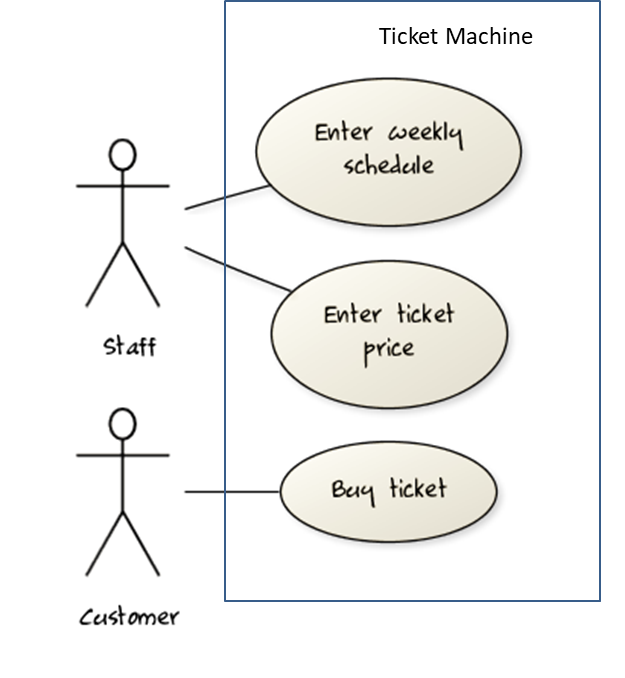#### Timing diagrams

Can explain timing diagrams

A timing diagram focuses on timing constraints.

Here is an example timing diagram: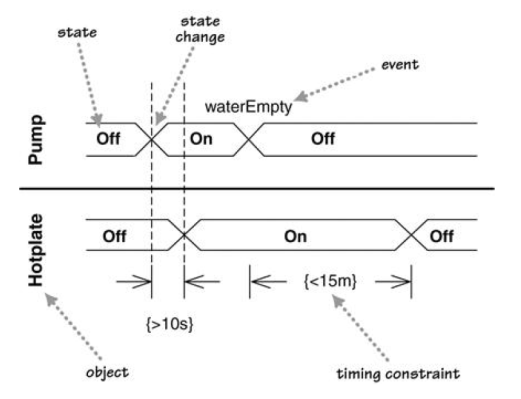Adapted from: UML Distilled by Martin Fowler

#### Interaction overview diagrams

Can explain interaction overview diagrams

Interaction overview diagrams are a combination of activity diagrams and sequence diagrams.

An example: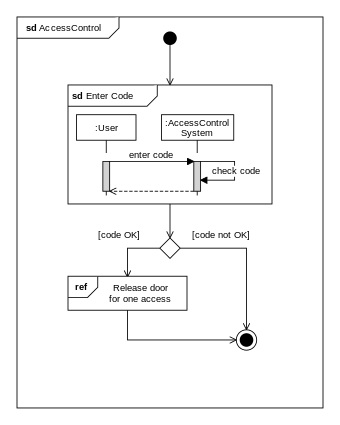source: https://commons.wikimedia.org

#### Communication diagrams

Can explain communication diagrams

Communication diagrams are like sequence diagrams but emphasize the data links between the various participants in the interaction rather than the sequence of interactions.

An example: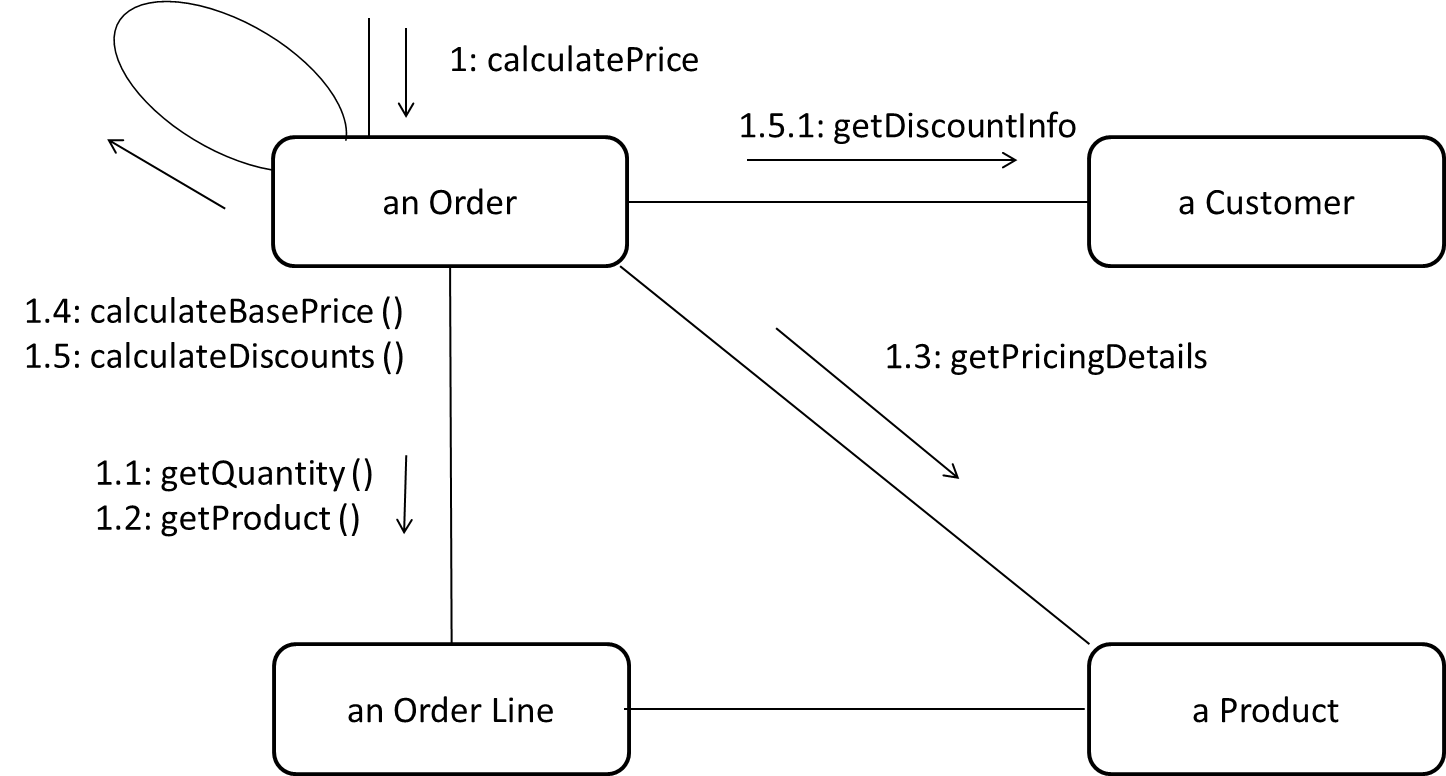Adapted from: UML Distilled by Martin Fowler

#### State machine diagrams

Can explain state machine diagrams

A State Machine Diagram models state-dependent behavior.

Consider how a CD player responds when the “eject CD” button is pushed:

• If the CD tray is already open, it does nothing.
• If the CD tray is already in the process of opening (opened half-way), it continues to open the CD tray.
• If the CD tray is closed and the CD is being played, it stops playing and opens the CD tray.
• If the CD tray is closed and CD is not being played, it simply opens the CD tray.
• If the CD tray is already in the process of closing (closed half-way), it waits until the CD tray is fully closed and opens it immediately afterwards.

What this means is that the CD player’s response to pushing the “eject CD” button depends on what it was doing at the time of the event. More generally, the CD player’s response to the event received depends on its internal state. Such a behavior is called a state-dependent behavior.

Often, state-dependent behavior displayed by an object in a system is simple enough that it needs no extra attention; such a behavior can be as simple as a conditional behavior like `if x > y, then x = x - y`.

Occasionally, objects may exhibit state-dependent behavior that is complex enough such that it needs to be captured in a separate model. Such state-dependent behavior can be modeled using UML state machine diagrams (SMD for short, sometimes also called ‘state charts’, ‘state diagrams’ or ‘state machines’).

An SMD views the life-cycle of an object as consisting of a finite number of states where each state displays a unique behavior pattern. SMDs capture information such as the states an object can be in during its lifetime, how the object responds to various events while in each state, and how the object transits from one state to another. In contrast to sequence diagrams that capture object behavior one scenario at a time, SMDs capture the object’s behavior over its full life-cycle.

An SMD for the Minesweeper game.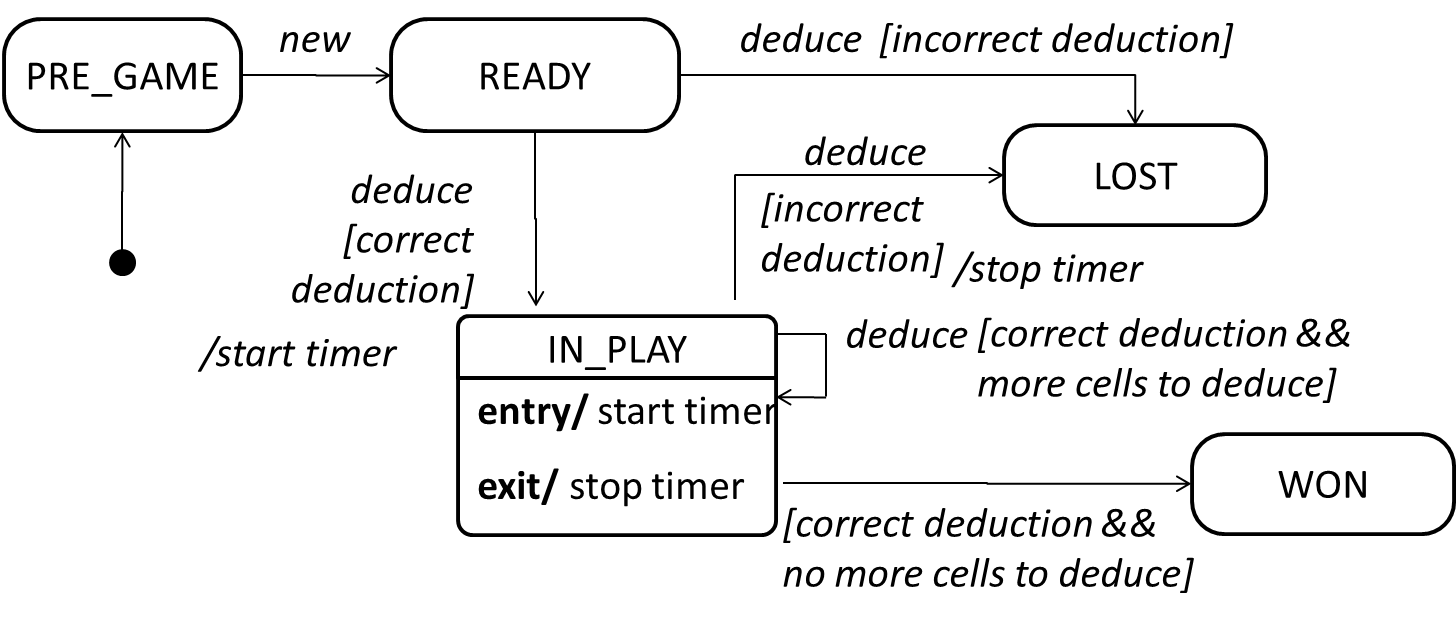## Modeling a solution

#### Introduction

Can explain how modeling can be used before implementation

You can use models to analyze and design software before you start coding.

Suppose you are planning to implement a simple minesweeper game that has a text based UI and a GUI. Given below is a possible OOP design for the game.Before jumping into coding, you may want to find out things such as,

• Is this class structure able to produce the behavior you want?
• What API should each class have?
• Do you need more classes?

To answer these questions, you can analyze how the objects of these classes will interact with each other to produce the behavior you want.

#### Basic

Can use simple class diagrams and sequence diagrams to model an OO solution

As mentioned in [Design → Modeling → Modeling a Solution → Introduction], this is the Minesweeper design you have come up with so far. Our objective is to analyze, evaluate, and refine that design.Let us start by modeling a sample interaction between the person playing the game and the `TextUi` object.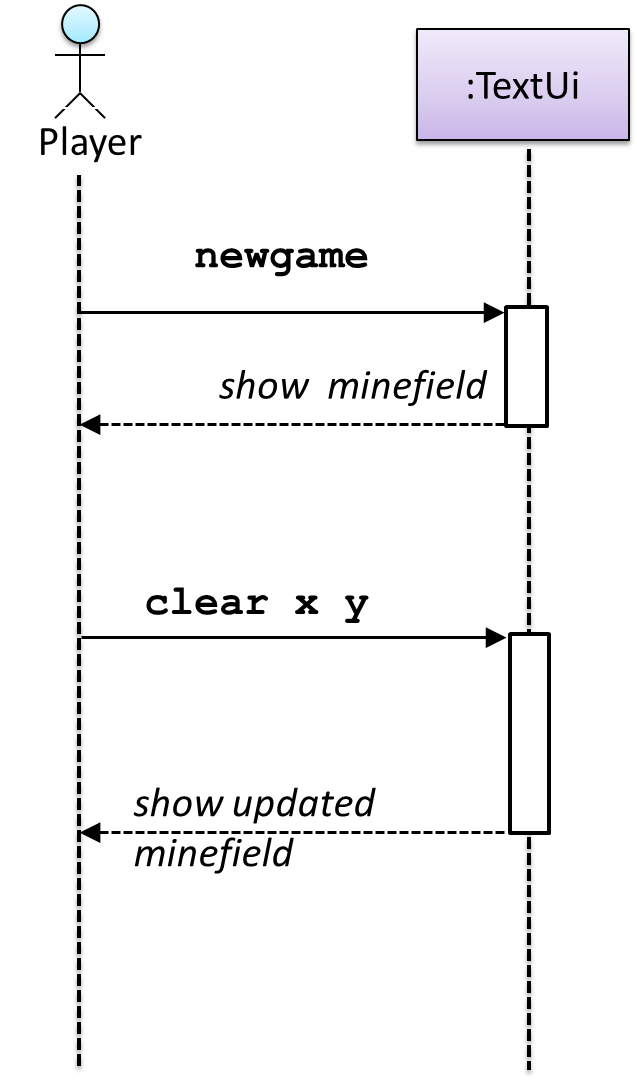`newgame` and `clear x y` represent commands typed by the `Player` on the `TextUi`.

How does the `TextUi` object carry out the requests it has received from the player? It would need to interact with other objects of the system. Because the `Logic` class is the one that controls the game logic, the `TextUi` needs to collaborate with `Logic` to fulfill the `newgame` request. Let us extend the model to capture that interaction.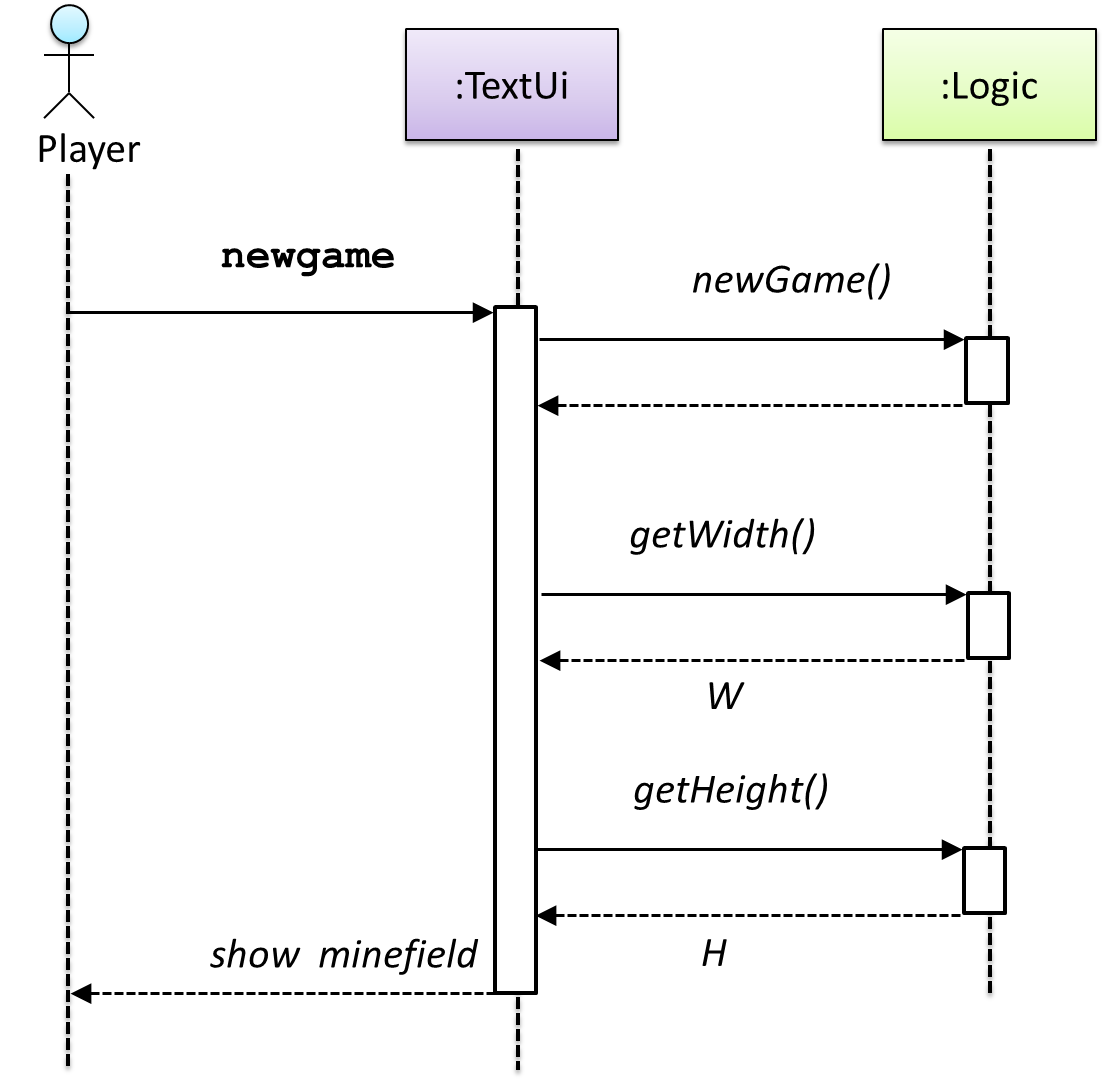`W` = Width of the minefield; `H` = Height of the minefield

The above diagram assumes that `W` and `H` are the only information `TextUi` requires to display the minefield to the `Player`. Note that there could be other ways of doing this.

The `Logic` methods you conceptualized in our modeling so far are: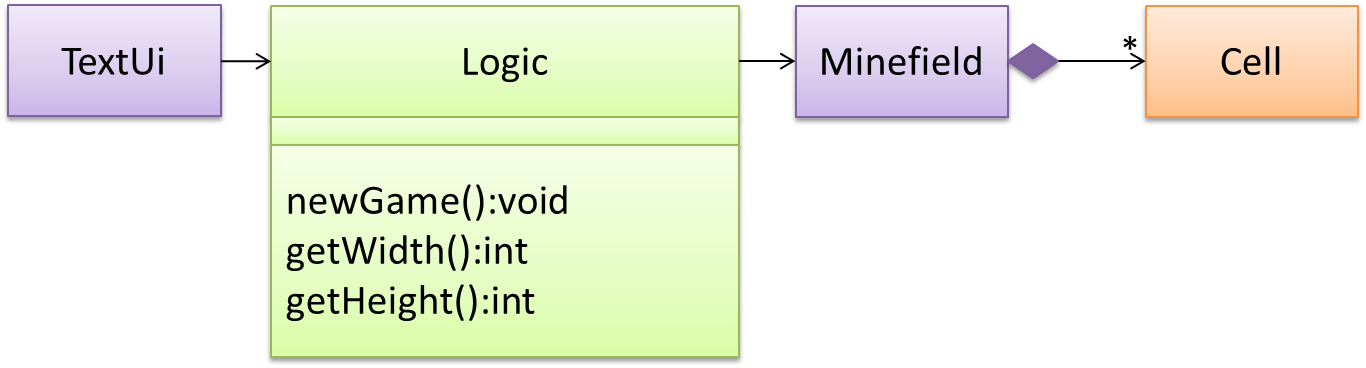Now, let us look at what other objects and interactions are needed to support the `newGame()` operation. It is likely that a new `Minefield` object is created when the `newGame()` method is called.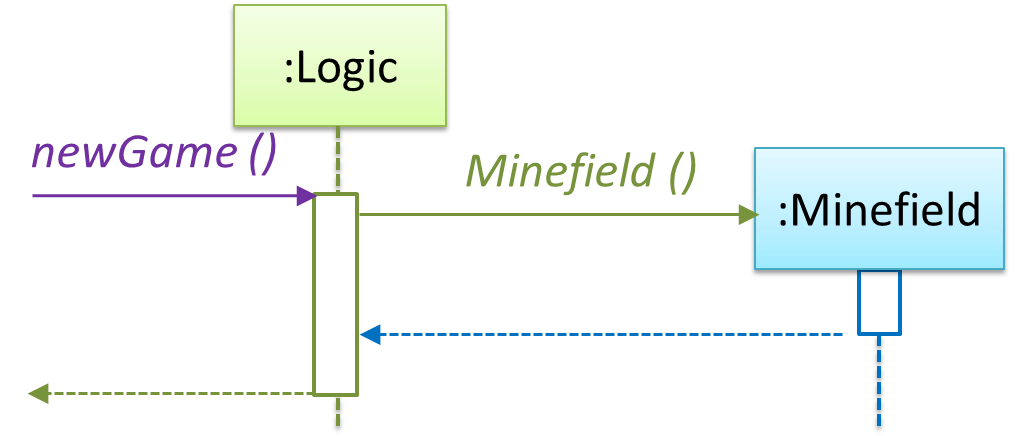Note that the behavior of the `Minefield` constructor has been abstracted away. It can be designed at a later stage.

Given below are the interactions between the player and the `TextUi` for the whole game.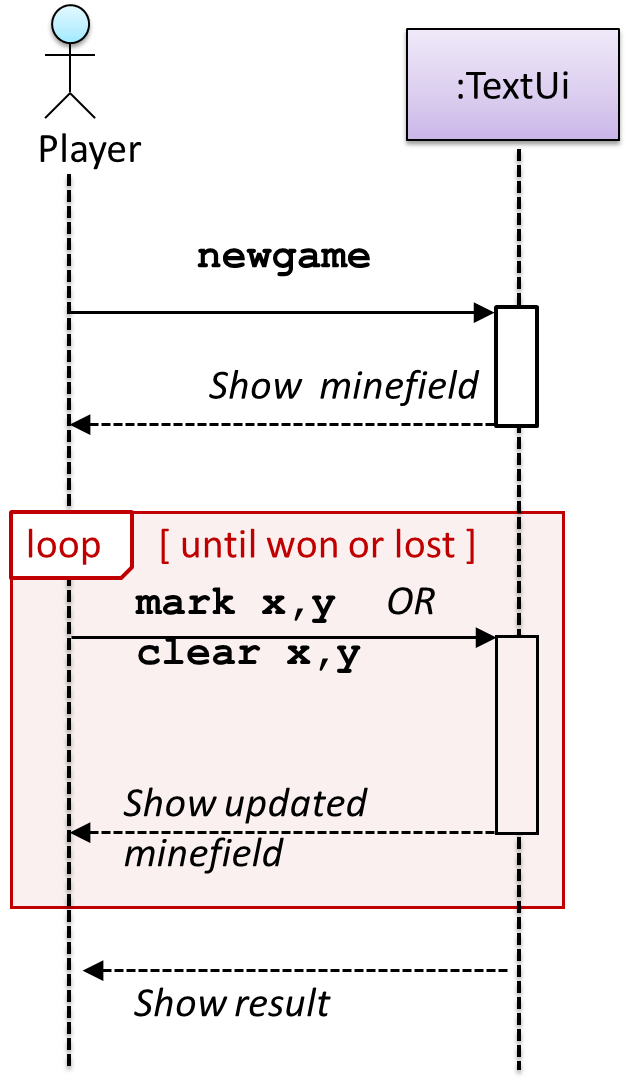Note that can be used when discovering/defining the architecture-level APIs.

Defining the architecture-level APIs for a small Tic-Tac-Toe game:

#### Intermediate

Can use intermediate class diagram and sequence diagram concepts to model an OO design

Continuing with the example in [Design → Modeling → Modeling a Solution → Basic], next let us model how the `TextUi` interacts with the `Logic` to support the mark and clear operations until the game is won or lost.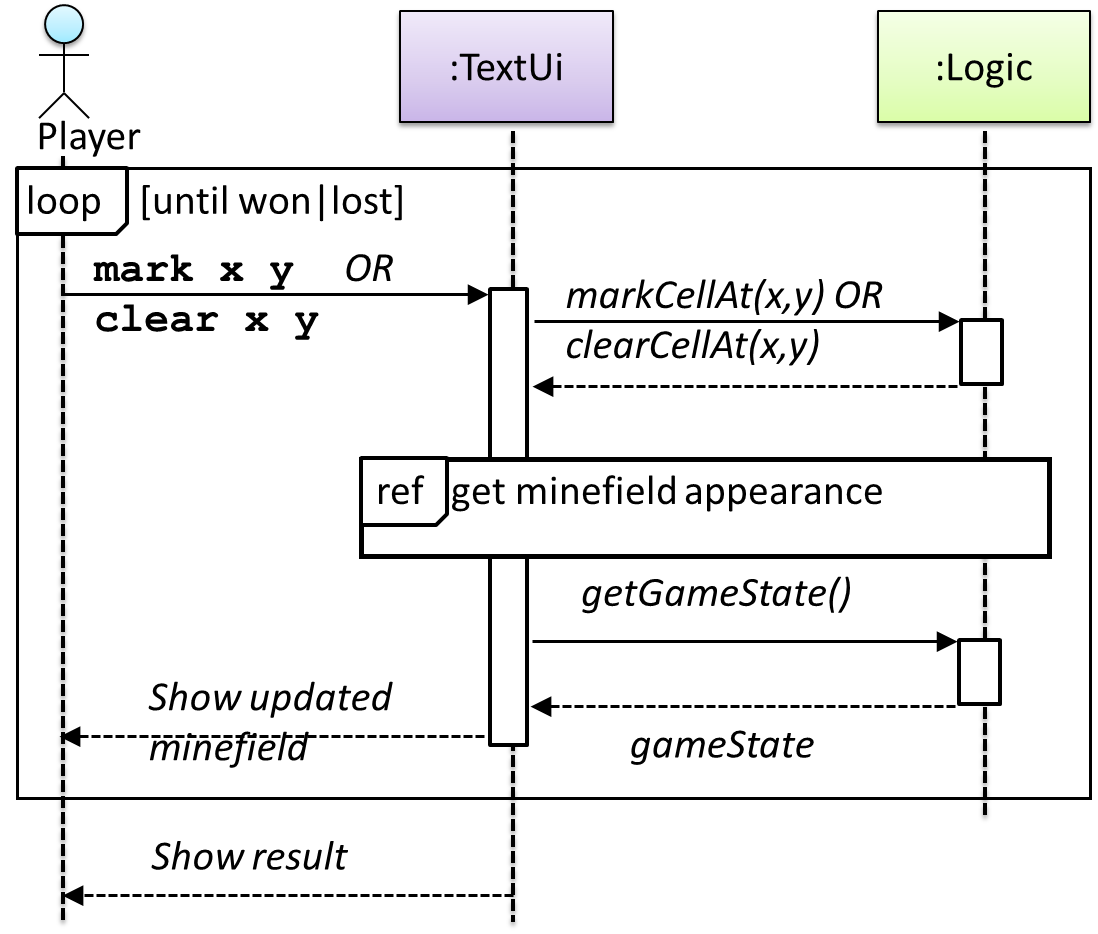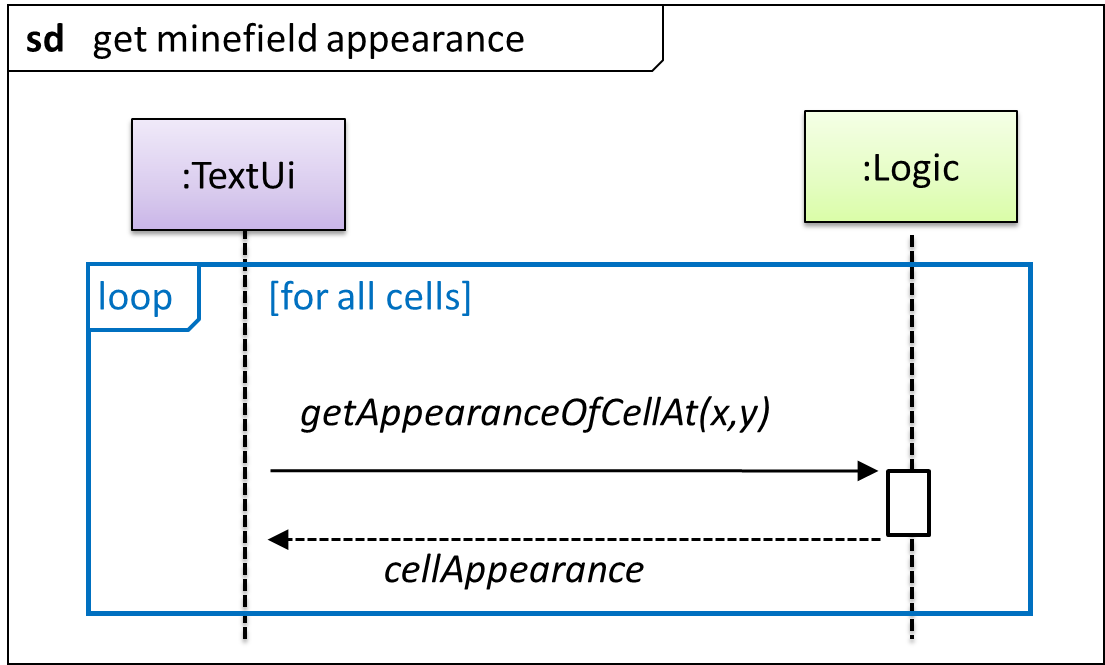This interaction adds the following methods to the `Logic` class:

• `clearCellAt(int x, int y)`
• `markCellAt(int x, int y)`
• `getGameState(): GAME_STATE (GAME_STATE: READY, IN_PLAY, WON, LOST, …)`

And it adds the following operation to Logic API:

• `getAppearanceOfCellAt(int,int): CELL_APPEARANCE (CELL_APPEARANCE: HIDDEN, ZERO, ONE, TWO, THREE, …, MARKED, INCORRECTLY_MARKED, INCORRECTLY_CLEARED)`

In the above design, `TextUi` does not access `Cell` objects directly. Instead, it gets values of type `CELL_APPEARANCE` from `Logic` to be displayed as a minefield to the player. Alternatively, each cell or the entire minefield can be passed directly to `TextUi`.

Here is the updated class diagram: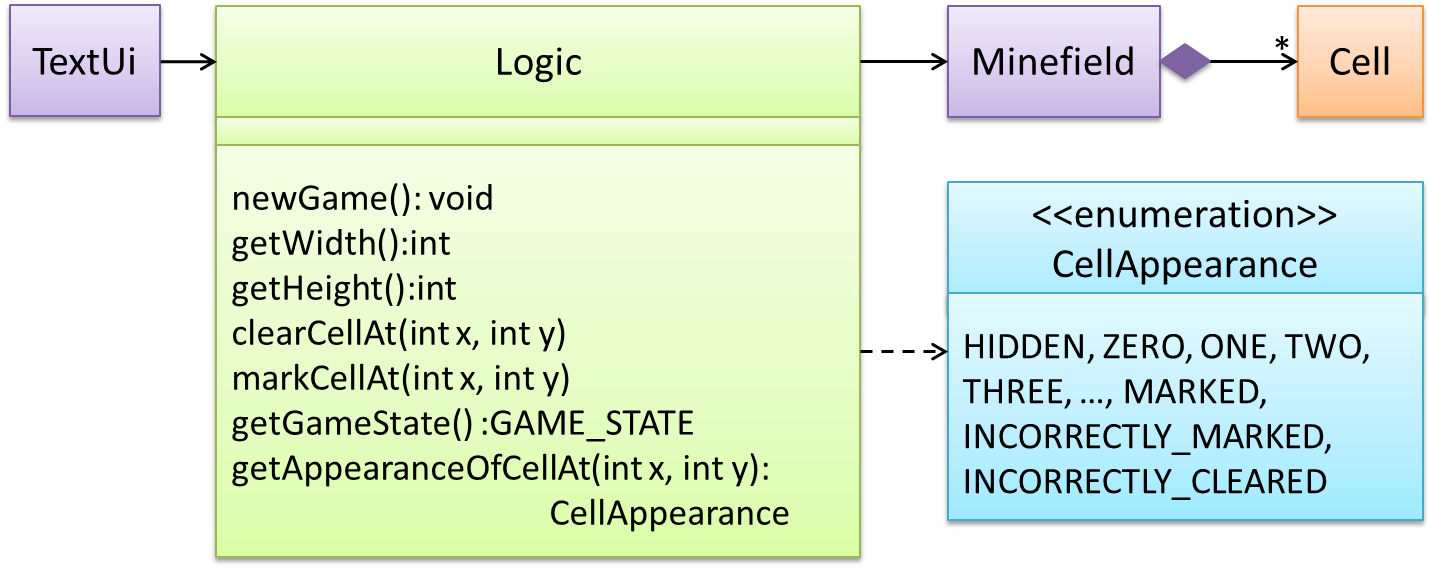The above is for the case when Actor `Player` interacts with the system using a text UI. Additional operations (if any) required for the GUI can be discovered similarly. Suppose `Logic` supports a `reset()` operation. You can model it like this: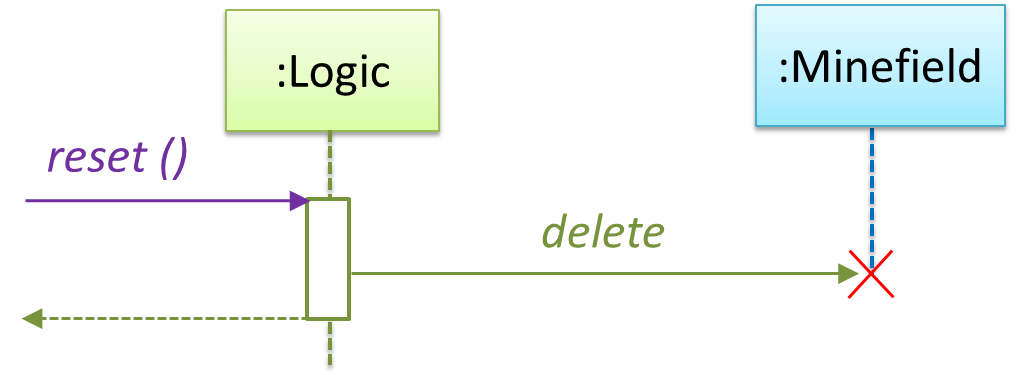Our current model assumes that the `Minefield` object has enough information (i.e. H, W, and mine locations) to create itself.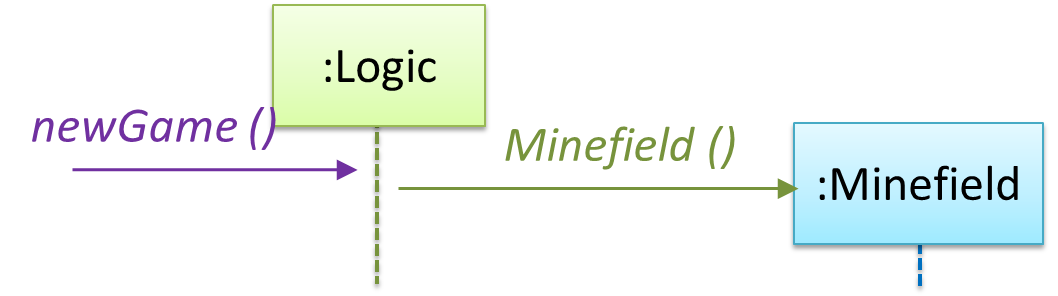An alternative is to have a `ConfigGenerator` object that generates a string containing the minefield information as shown below.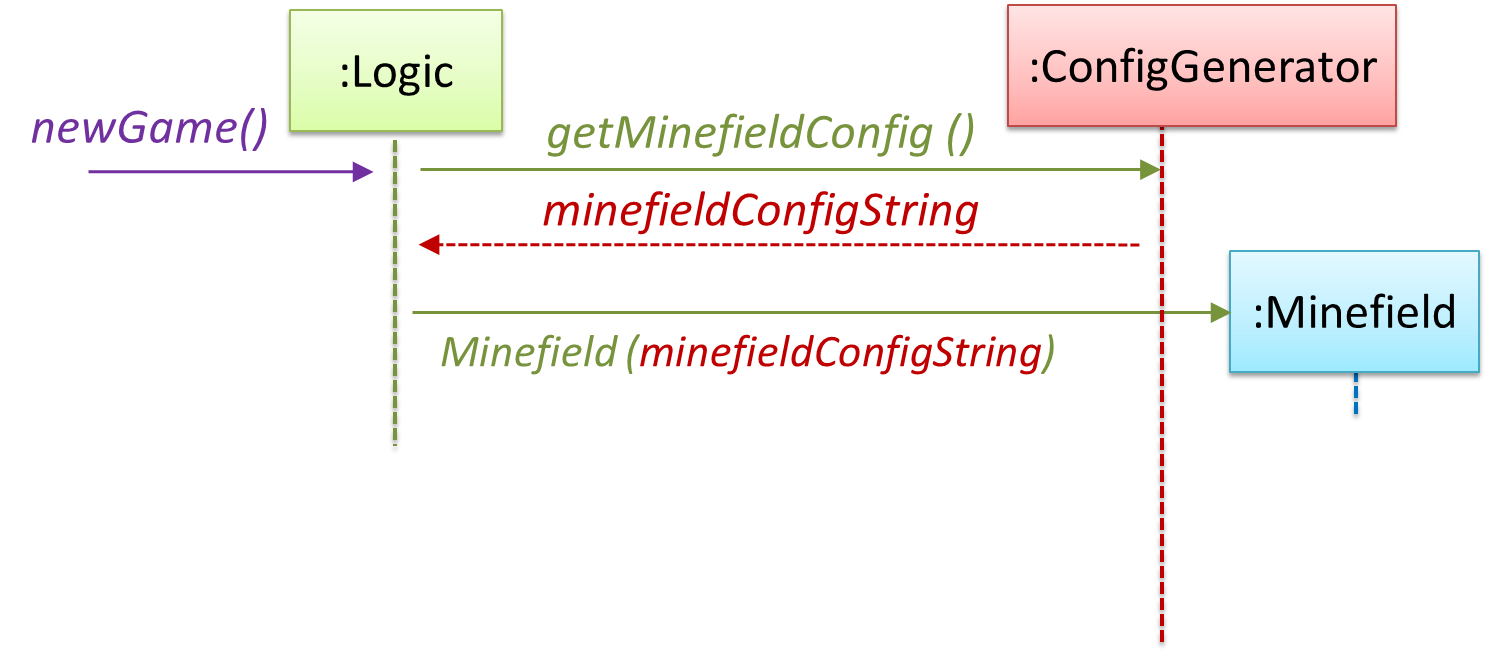In addition, `getWidth()`, `getHeight()`, `markCellAt(x,y)` and `clearCellAt(x,y)` can be handled like this.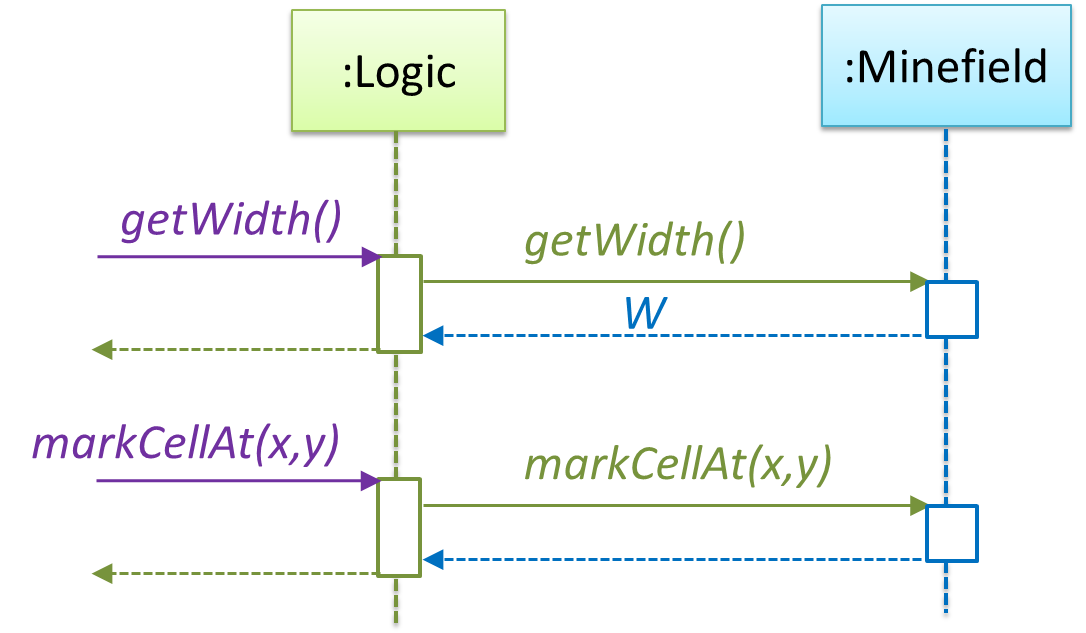The updated class diagram: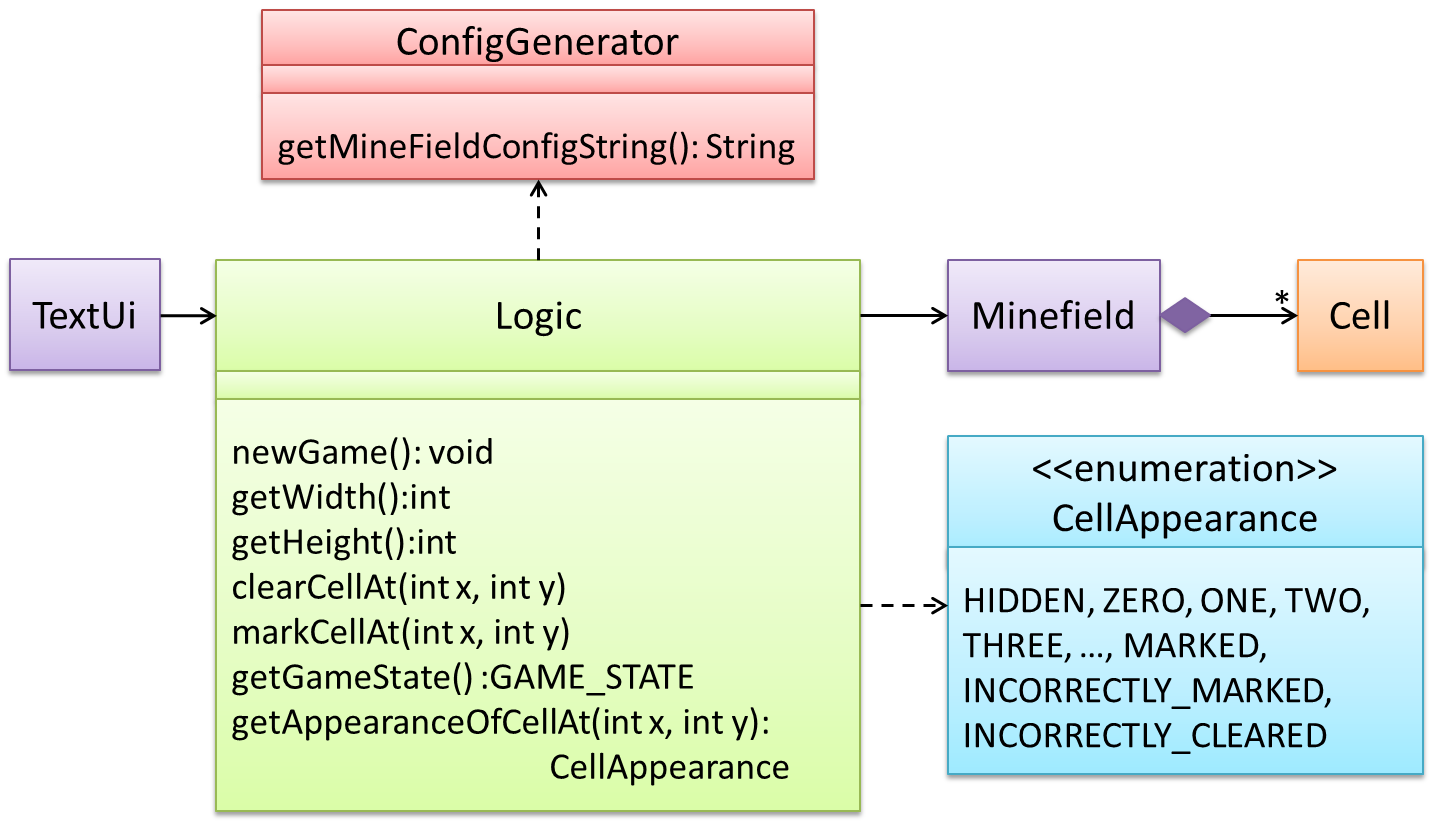How is the `getGameState()` operation supported? Given below are two ways (there could be other ways):

1. The `Minefield` class knows the state of the game at any time. The `Logic` class retrieves it from the `Minefield` class as and when required.
2. The `Logic` class maintains the state of the game at all times.

Here’s the SD for option 1.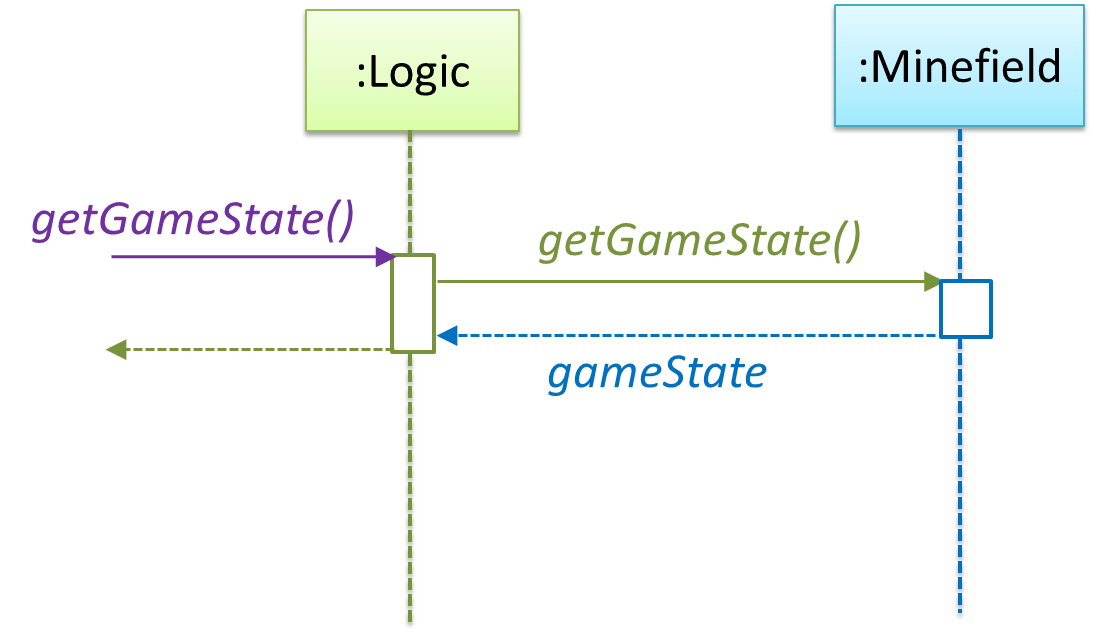Here’s the SD for option 2. Assume that the game state is updated after every mark/clear action.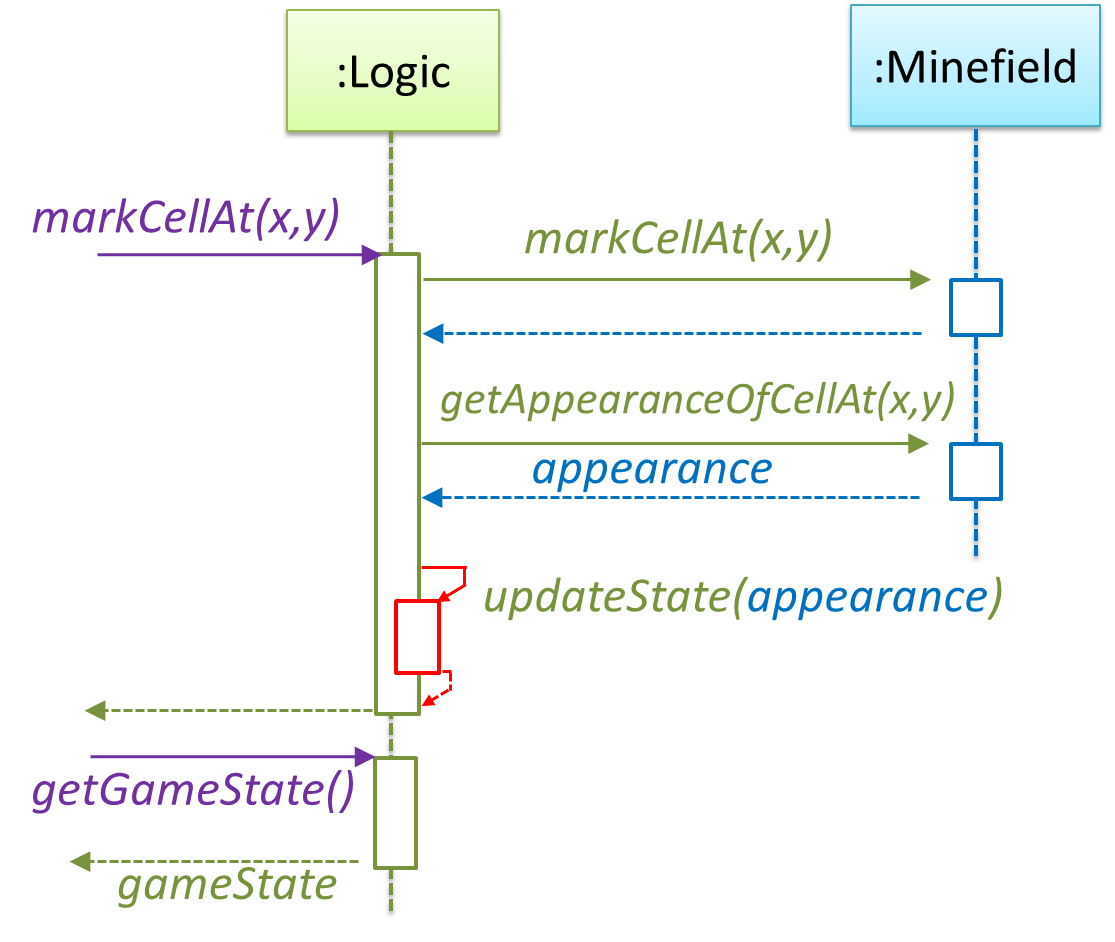It is now time to explore what happens inside the `Minefield` constructor. One way is to design it as follows.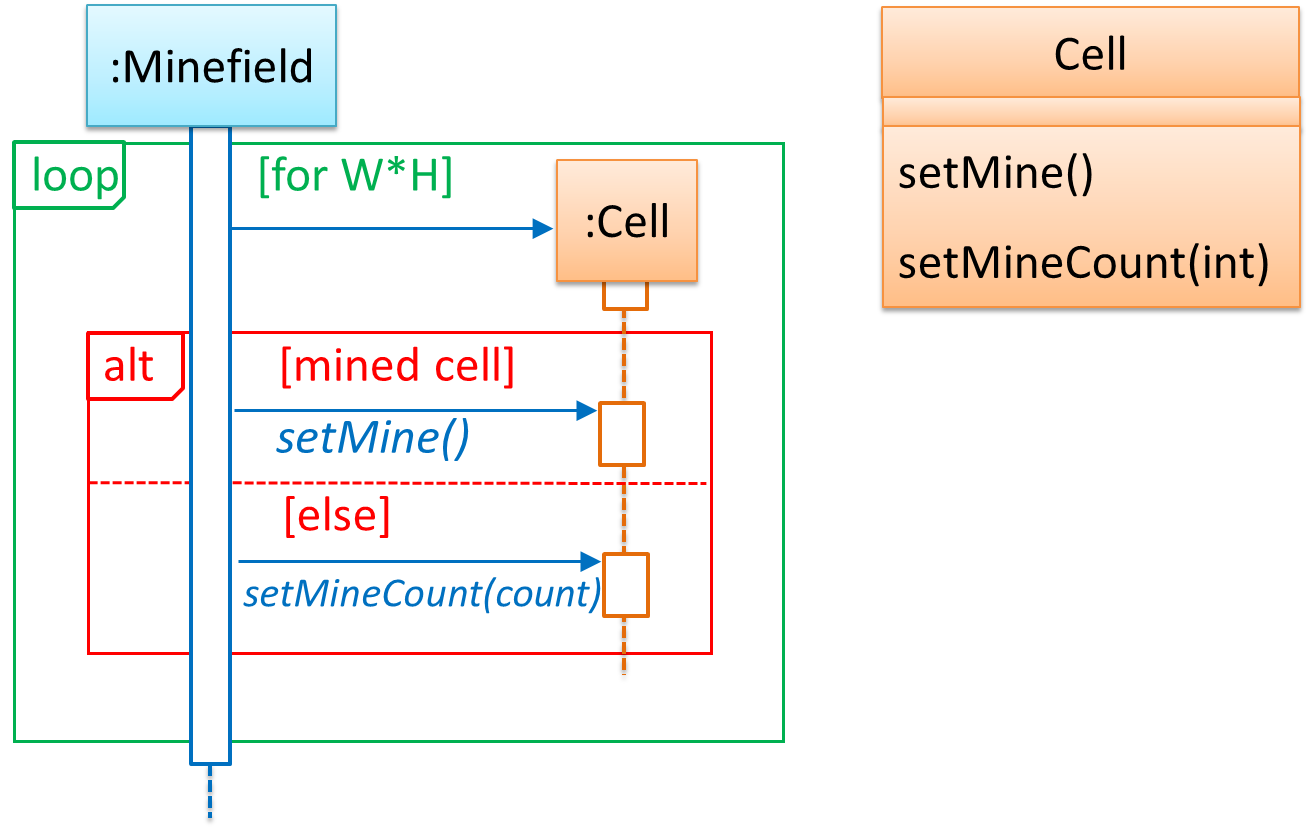Now let us assume that `Minesweeper` supports a ‘timing’ feature.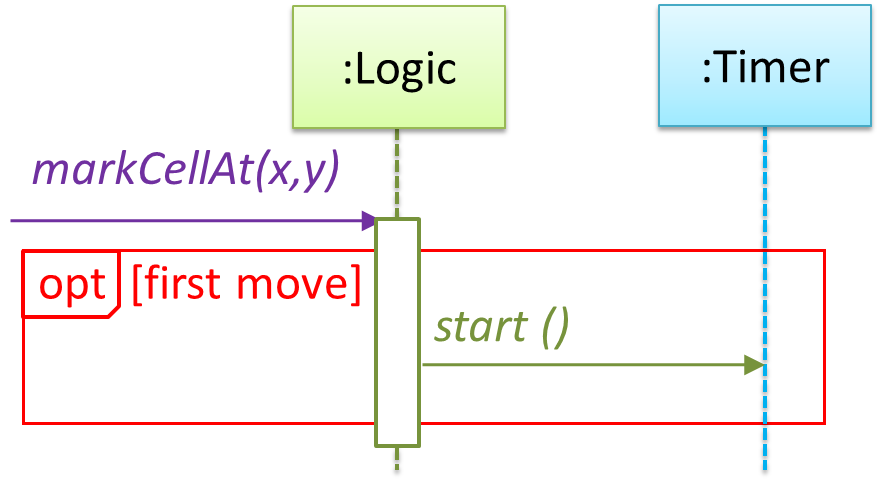Updated class diagram: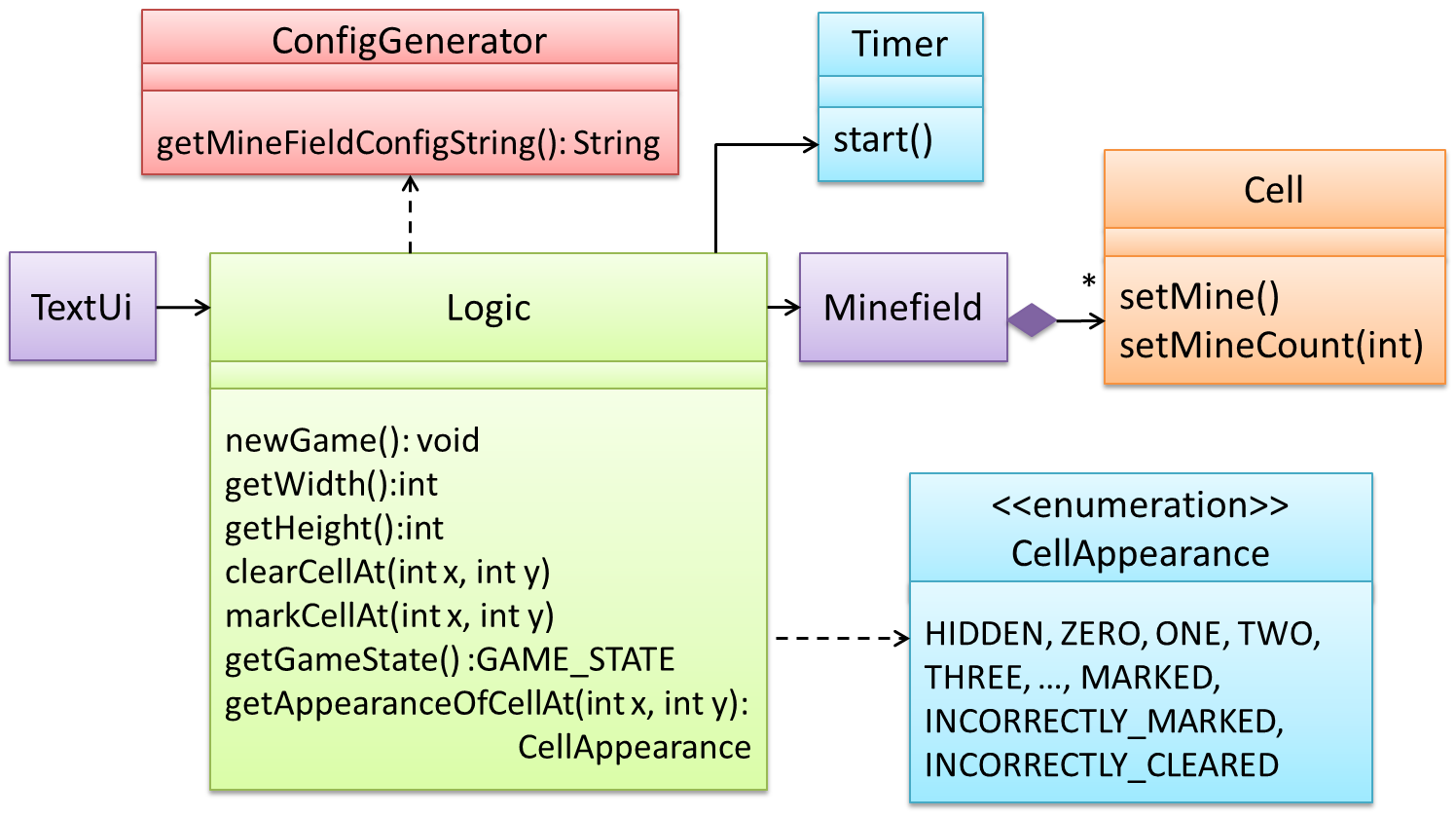When designing components, it is not necessary to draw elaborate UML diagrams capturing all details of the design. They can be done as rough sketches. For example, draw sequence diagrams only when you are not sure which operations are required by each class, or when you want to verify that your class structure can indeed support the required operations.